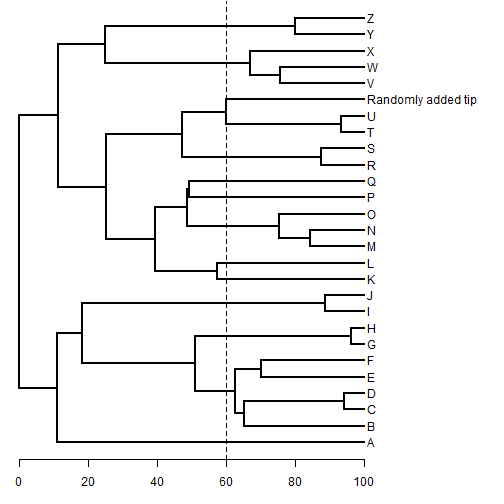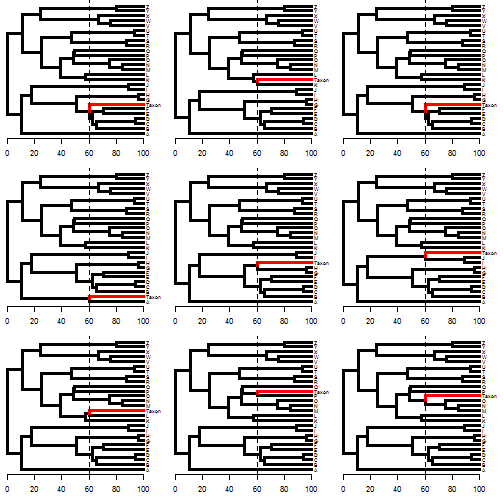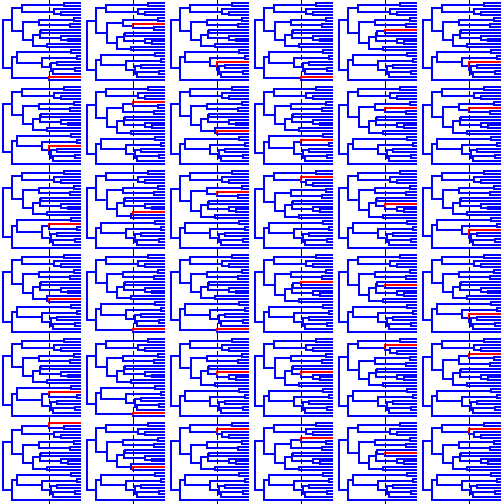## Monday, April 18, 2016

### Adding a tip at random, but at a fixed height or depth

A phytools user/reader recently posted the following question:

“In the `add.random` function, using an ultrametric tree, is there any way to control the edge lengths? Ideally I wanna constrain where (timewise) the new tips get added. I don't care where they end up relationship- wise, just want to be able to add them all say <10 million years, or <5 million years, what have you."

There is not a way to do this automatically within `add.random`, but it is fairly straightforward with `bind.tip`.

Here I deal with the (fairly) straightforward problem of adding a tip randomly to a tree at a fixed depth. Perhaps in another post I will address the issue of adding a tip on a time interval.

``````library(phytools)
## for our purposes, simulate a tree of depth 100
tree<-pbtree(n=26,tip.label=LETTERS,scale=100)
## add a tip randomly with height above the root of 60:
h<-60
H<-nodeHeights(tree)
ii<-intersect(which(H[,1]<h),which(H[,2]>h))
edges<-tree\$edge[ii,2]
depths<-H[ii,2]-h
## pick one at random:
jj<-sample(1:length(edges),1)
plotTree(obj,mar=c(3.1,0.1,0.1,0.1))
axis(1,at=seq(0,100,by=20))
lines(rep(h,2),par()\$usr[3:4],lty="dashed")
``````We can write a silly little function to repeat this, though here I will give it as "depth” (from the present), rather than height above the root:

``````bind.at.depth<-function(tree,tip.label,depth){
H<-nodeHeights(tree)
h<-max(H)-depth
ii<-intersect(which(H[,1]<h),which(H[,2]>h))
edges<-tree\$edge[ii,2]
depths<-H[ii,2]-h
jj<-sample(1:length(edges),1)
bind.tip(tree,tip.label,where=edges[jj],position=depths[jj])
}
``````

and then replicate it a bunch of times. Here, when I plot them, for fun I'll paint the added edge red:

``````trees<-replicate(9,bind.at.depth(tree,"Taxon",40),simplify=FALSE)
class(trees)<-"multiPhylo"
par(mfrow=c(3,3))
for(i in 1:9){
plot(paintBranches(trees[[i]],which(trees[[i]]\$tip.label=="Taxon"),
state="2"),mar=c(3.1,0.1,0.1,0.1),fsize=0.6,lwd=3,
split.vertical=TRUE)
axis(1,at=seq(0,100,by=20))
lines(rep(60,2),par()\$usr[3:4],lty="dashed")
}
``````
``````## no colors provided. using the following legend:
##       1       2
## "black"   "red"
``````
``````## no colors provided. using the following legend:
##       1       2
## "black"   "red"
``````
``````## no colors provided. using the following legend:
##       1       2
## "black"   "red"
``````
``````## no colors provided. using the following legend:
##       1       2
## "black"   "red"
``````
``````## no colors provided. using the following legend:
##       1       2
## "black"   "red"
``````
``````## no colors provided. using the following legend:
##       1       2
## "black"   "red"
``````
``````## no colors provided. using the following legend:
##       1       2
## "black"   "red"
``````
``````## no colors provided. using the following legend:
##       1       2
## "black"   "red"
``````
``````## no colors provided. using the following legend:
##       1       2
## "black"   "red"
``````Or, with even more replicates!

``````trees<-replicate(36,bind.at.depth(tree,"Taxon",40),simplify=FALSE)
class(trees)<-"multiPhylo"
par(mfrow=c(6,6))
for(i in 1:36){
plot(paintBranches(trees[[i]],which(trees[[i]]\$tip.label=="Taxon"),
state="2"),ftype="off",lwd=2,split.vertical=TRUE,
colors=setNames(c("blue","red"),1:2))
lines(rep(60,2),par()\$usr[3:4],lty="dashed")
}
``````That's all there is to it!

1.Thanks so much for this script Liam.
I pirated it a bit by using your bind.at.depth function in a loop, to add a number of tips, branching at the same time, to the same tree:

species<-c(1:10)
for(i in 1:length(species)) tree<-bind.at.depth(tree, "taxon",2)
plot(tree)

Cheers.
Ian

2.Hi Liam,
This was incredibly helpful thank you! I have a question about how you might reduce the range of addition sites (place the taxa randomly within a subfamily/genus etc. at a specific age). I'm running to the problem of changing node numbers when adding multiple taxa. Any suggestions?
Thank,
Michael

Note: due to the very large amount of spam, all comments are now automatically submitted for moderation.